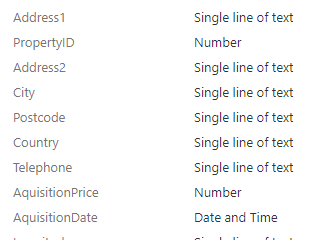# Data - The most effective ways to get a random record or row from a data source

If you want to select a random row or record from a data source, this post summarises the syntax that we can use.

There are often times where it's necessary to slect one or more random records from in the data source. Some example use case scenarios could include:

• Displaying a random record on a dashboard type screen
• Retrieving a random record for gaming type apps (eg, retrieving a random question from a question table)
• For task allocation apps, selecting a random employee to allocate to a job

## The easiest way to select a random record

The easiest way to select a random record from a data source is to call the Shuffle function. This function takes an input table or data source, and returns a table of randomised records.

To demonstrate the use of this function, let's take a SharePoint list of property records. The schema of this list is shown beneath:To retrieve a single random record from this list, we can add a button, retrieve the first record from the Shuffle function, and assign the result to a variable (varRecord). The formula we would use looks like this:

`Set(varRecord,    First(Shuffle(Property)))`

To display the random record, we can add a display form to a screen, and set the item property to varRecord.

## What's the problem with the Shuffle function?

The shuffle function is very simple to use, but the problem is that it is not delegable. To illustrate this behaviour, let's set the "data row limit" of our app to 10. If we monitor our app and retrieve a random record, we see that Power Apps retrieves 10 rows from the data source and returns a random record from this subset of 10 rows.## How to workaround the delegation limit of Shuffle

In many cases, it's important to be able to select a random record from an entire table or SharePoint list. If our input data source contains some sort of numeric identity column, we can use this to help return a random record.

We would call the Rand() function to generate a random number that's equal to or less than the highest numeric value, and to select the first record that exceeds the random number.

In this example, our SharePoint list includes a "PropertyID" column which stores a unique numeric identifier for each record.

The formula to return a random record would look like this:

`With({lastID:First(FirstN(SortByColumns(Property, "PropertyID",Descending),1)).PropertyID},      With({randomID:lastID * Rand()},                          Set(varRecord, First(FirstN(Filter(Property, PropertyID >= randomID ),1)))))`

The first part of this formula retrieves the maximum PropertyID value using the First/FirstN pattern to retrieve the value in the most efficient way. Next, we multiply this highest value by the output of the Rand() function to generate a random number less than the maximum property id value.

If we were to attach this formula to a button, the user can now click the button to assign a random record to varRecord in a delegable way.

## Conclusion

A simple way to select a random record from a data source is to call the Shuffle function. This function is simple to use, but it is not delegable, meaning that it won't return random records from data sources that exceed 2,000 records (or the data row limit value). For data sources with a unique numeric identifier, one way to workaround this limitation is to calculate a random number, and to select the first record equal to or greater than this random number.